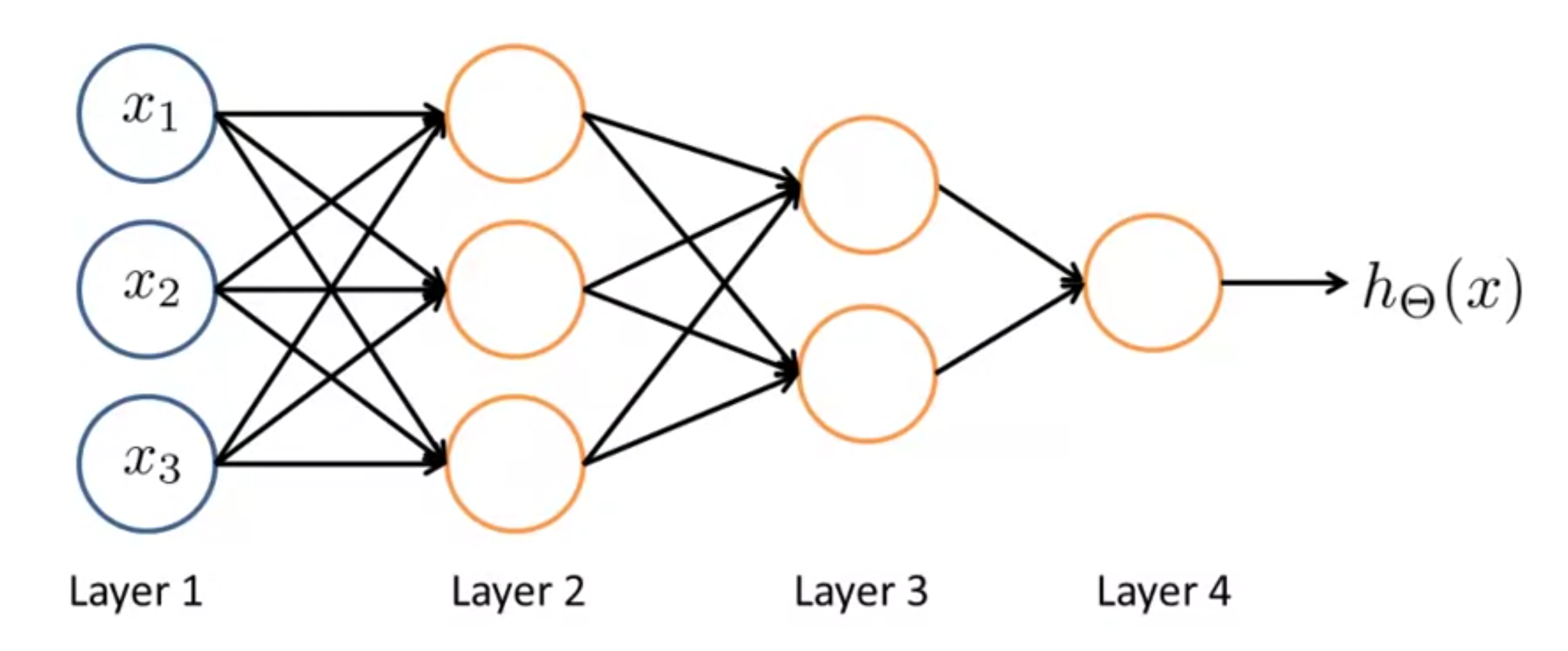Neural networks were a lot easier to understand than I thought - at least those used for classification that I’ve learned about so far. They’re essentially a network of binary switches that outputs a final classification. It reminds me of a multi-pole light switch set up.

These types of neural networks can be created to form any logical operations: OR, AND, NOT AND (NAND), EXCLUSIVE OR (XOR).

Each layer uses the same Sigmoid function from logistic regression classification. Each target in the network represents an activation function that takes the previous layer node outputs as inputs weighted by a separate matrix of parameters, $$\ \Theta$$ (with a capital T).

Each layer also adds a bias node, $$a_{0}^{(i)} = 1$$ where $$\ i$$ is the layer of the network.

The neural network is comprised of three types of layers: an input layer comprised of your input variables, an output layer comprised of your final activation function and which outputs the value of your hypothesis, and an unlimited number of hidden layers between them.